Electron. J. Diff. Equ., Vol. 2011 (2011), No. 64, pp. 1-22.

### Weighted eigenvalue problems for the p-Laplacian with weights in weak Lebesgue spaces T. V. Anoop

Abstract:
We consider the nonlinear eigenvalue problem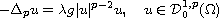where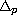is the p-Laplacian operator,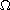is a connected domain in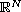with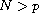and the weight function g is locally integrable. We obtain the existence of a unique positive principal eigenvalue for g such that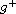lies in certain subspace of weak-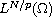. The radial symmetry of the first eigenfunctions are obtained for radial g, whenis a ball centered at the origin or. The existence of an infinite set of eigenvalues is proved using the Ljusternik-Schnirelmann theory on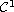manifolds.

Submitted November 11, 2010. Published May 17, 2011.
Math Subject Classifications: 35J92, 35P30, 35A15.
Key Words: Lorentz spaces; principal eigenvalue; radial symmetry; Ljusternik-Schnirelmann theory.

Show me the PDF file (360 KB), TEX file, and other files for this article.T. V. Anoop The Institute of Mathematical Sciences Chennai 600113, India email: tvanoop@imsc.res.in